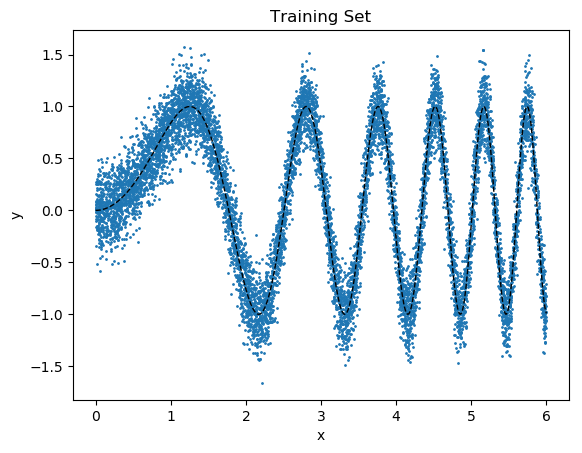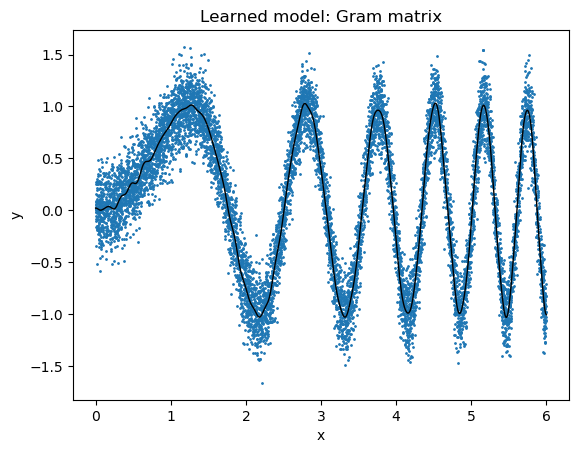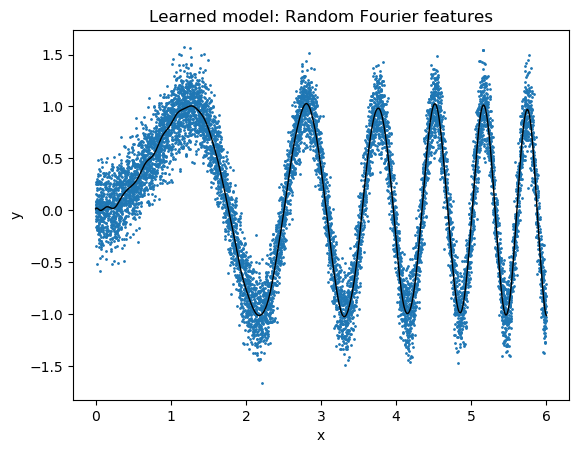# Kernel learning example¶

Here, we imagine that we want to use linear regression with a Gaussian kernel to fit a noisy sine wave.

In :
using PyPlot
using Random
using LinearAlgebra
import Statistics.mean

In :
Random.seed!(123456);
N = 10000; # size of data set
x = 6 * rand(N);
y = sin.(x.^2) .+ 0.2 * randn(N);

In :
scatter(x,y; s=1);
plot(collect(0:0.01:6), sin.(collect(0:0.01:6).^2), "--k"; linewidth=1);
xlabel("x");
ylabel("y");
title("Training Set");In :
gamma = 100;
function K(x1, x2)
return exp(-gamma*(x1 - x2)^2/2);
end

Out:
K (generic function with 1 method)
In :
# compute the Gram matrix
@time begin
G = zeros(N, N);
for i = 1:N
for j = 1:N
G[i,j] = K(x[i], x[j]);
end
end
end

 20.894899 seconds (889.82 M allocations: 15.495 GiB, 3.97% gc time)

In :
# actually solve the regularized linear regression problem
@time w = (G + 0.001 * LinearAlgebra.I) \ y;

  6.024515 seconds (4.35 M allocations: 1.696 GiB, 2.65% gc time)

In :
function predict(xt :: Float64)
rv = 0.0;
for i = 1:N
rv += w[i] * K(x[i], xt);
end
return rv;
end

Out:
predict (generic function with 1 method)
In :
scatter(x,y; s=1);
x_test = collect(0:0.01:6);
y_test = sin.(x_test.^2);
y_pred = predict.(x_test);
plot(x_test, y_pred, "k"; linewidth=1);
xlabel("x");
ylabel("y");
title("Learned model: Gram matrix");

println("average test error: $(sqrt(mean((y_test - y_pred).^2)))")average test error: 0.01885103613764682  ## Now, what if we do it with random Fourier features?¶ In : D = 1000; U = randn(D) * sqrt(gamma); b = 2 * pi * rand(D);  In : # compute the feature map @time begin phi = zeros(N, D); for i = 1:N for j = 1:D phi[i,j] = sqrt(2)*cos(U[j] * x[i] + b[j]); end end end   2.297546 seconds (99.89 M allocations: 1.714 GiB, 9.84% gc time)  In : # actually solve the (now not regularized) linear regression problem @time w_rff = (phi' * phi + 0.001 * LinearAlgebra.I) \ (phi' * y);   0.444575 seconds (1.13 M allocations: 79.020 MiB, 3.10% gc time)  In : function predict_rff(xt :: Float64) phi_xt = sqrt(2)*cos.(U .* xt .+ b); return dot(phi_xt, w_rff); end  Out: predict_rff (generic function with 1 method) In : scatter(x,y; s=1); x_test = collect(0:0.01:6); y_test = sin.(x_test.^2); y_pred = predict_rff.(x_test); plot(x_test, y_pred, "k"; linewidth=1); xlabel("x"); ylabel("y"); title("Learned model: Random Fourier features"); println("average test error:$(sqrt(mean((y_test - y_pred).^2)))")average test error: 0.01543744268322504

In [ ]:


In [ ]: